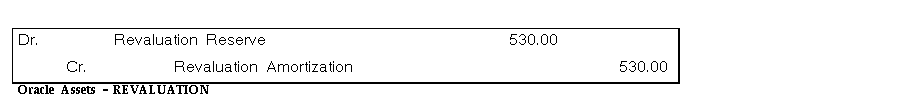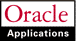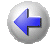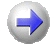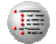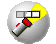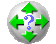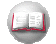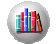Previous Next Contents Index Navigation Glossary Library

## Journal Entries for Revaluations

The following examples illustrate the effect on your assets and your accounts when you specify different revaluation rules.

### Revalue Accumulated Depreciation

Example 1: You place an asset in service in Year 1, Quarter 1. The asset cost is \$10,000, the life is 5 years, and you are using straight-line depreciation.

In Year 2, Quarter 1 you revalue the asset using a revaluation rate of 5%. Then in Year 4, Quarter 1 you revalue the asset again using a revaluation rate of -10%.

Revaluation Rules:

• Revalue Accumulated Depreciation = Yes
• Amortize Revaluation Reserve = No
• Retire Revaluation Reserve = No
Oracle Assets bases the new depreciation expense on the revalued remaining net book value.

In Year 5, Quarter 4, at the end of the asset's life, you retire the asset with no proceeds of sale or cost of removal.

The effects of the revaluations are illustrated in the following table: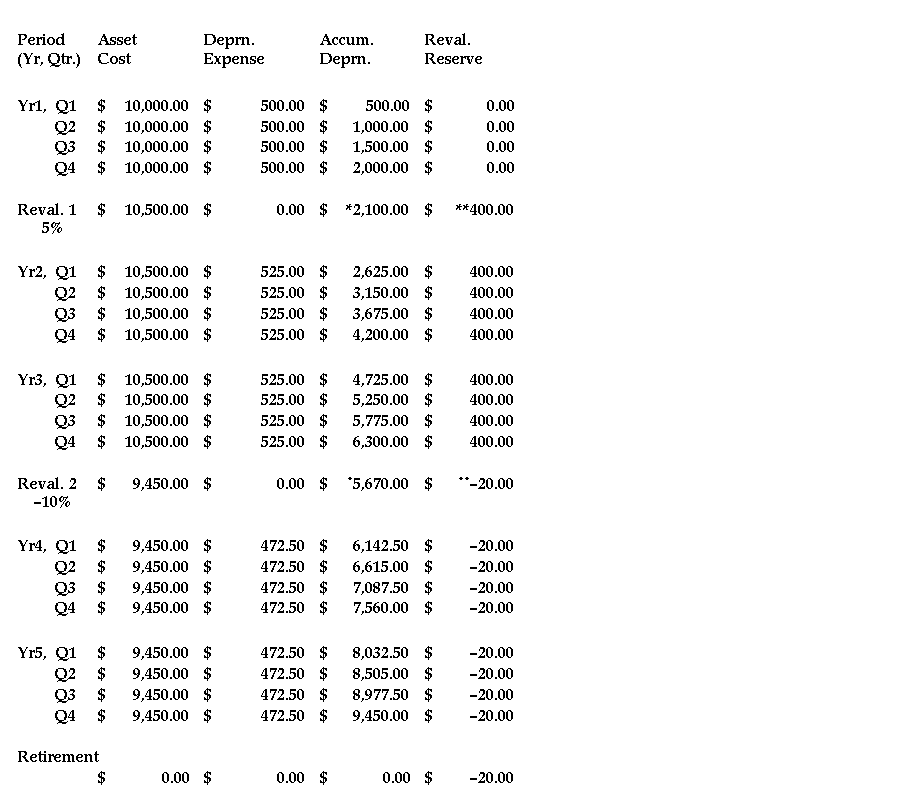#### REVALUATION 1

Year 2, Quarter 1, 5% revaluation#### REVALUATION 2

-10% revaluation in Year 4, Quarter 1: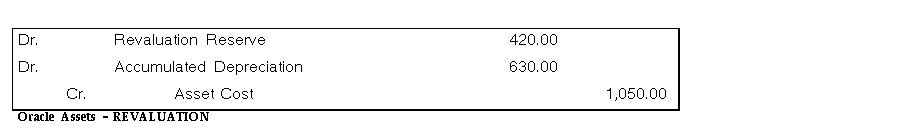Retirement in Year 5, Quarter 4: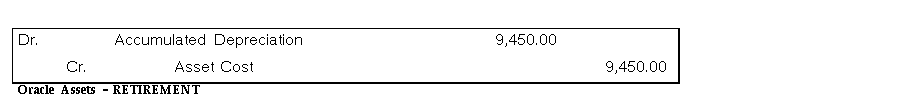### Accumulated Depreciation Not Revalued

Example 2: You place an asset in service in Year 1, Quarter 1. The asset cost is \$10,000, the life is 5 years, and you are using straight-line depreciation.

In Year 2, Quarter 1 you revalue the asset using a revaluation rate of 5%. Then in Year 4, Quarter 1 you revalue the asset again using a revaluation rate of -10%.

Revaluation Rules:

• Revalue Accumulated Depreciation = No
• Amortize Revaluation Reserve = No
• Retire Revaluation Reserve = Yes
For the first revaluation, the asset's new revalued cost is \$10,500. Since you do not revalue the accumulated depreciation, Oracle Assets transfers the balance to the revaluation reserve in addition to the change in cost.

Since you are also not amortizing the revaluation reserve, this amount remains in the revaluation reserve account until you retire the asset, when Oracle Assets transfers it to the appropriate revaluation reserve retired account. Oracle Assets bases the new depreciation expense on the revalued net book value.

For the second revaluation, the asset's revalued cost is \$9,450. Again, since you do not revalue the accumulated depreciation, Oracle Assets transfers the balance to the revaluation reserve along with the change in cost.

You retire the asset in Year 5, Quarter 4, with no proceeds of sale or cost of removal.

The effects of the revaluations are illustrated in the following table: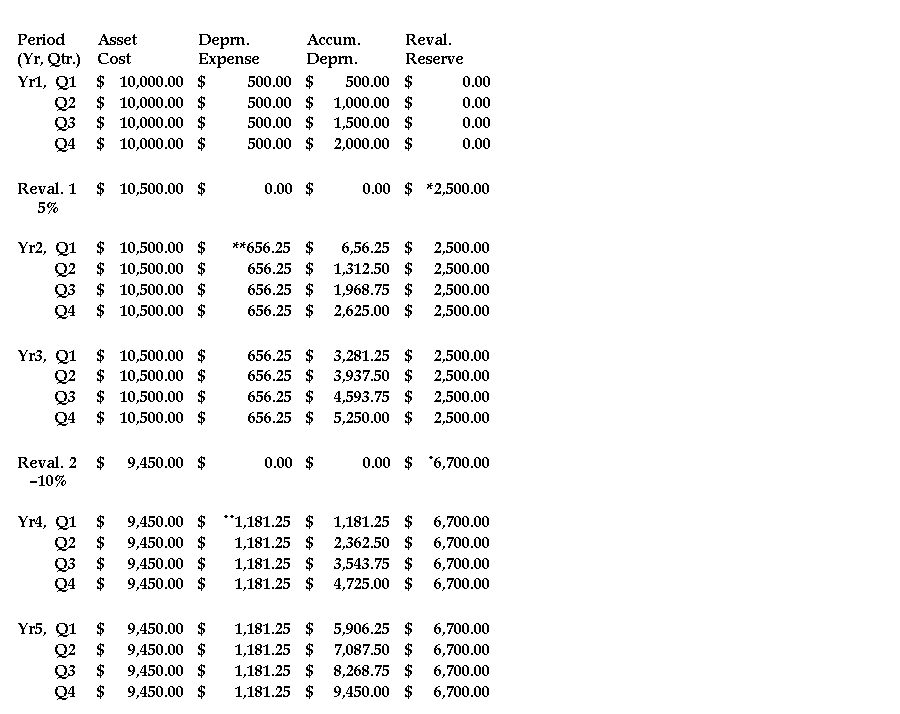#### REVALUATION 1

5% revaluation in Year 2, Quarter 1: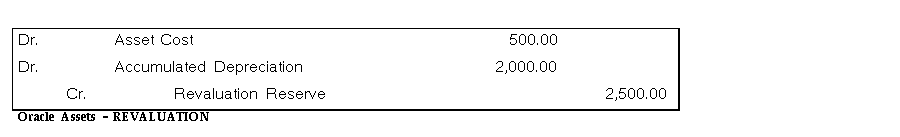#### REVALUATION 2

-10% revaluation in Year 4, Quarter 1: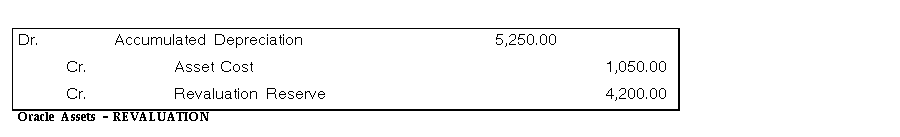Retirement in Year 5, Quarter 4: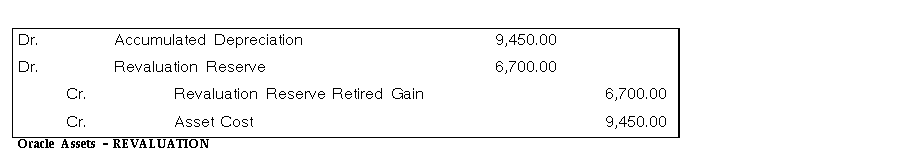### Amortizing Revaluation Reserve

Example 3: You place an asset in service in Year 1, Quarter 1. The asset cost is \$10,000, the life is 5 years, and you are using straight-line depreciation.

In Year 2, Quarter 1 you revalue the asset using a rate of 5%. Then in Year 4, Quarter 1 you revalue the asset again using a rate of -10%.

Revaluation Rules:

• Revalue Accumulated Depreciation = No
• Amortize Revaluation Reserve = Yes
For the first revaluation, the asset's new revalued cost is \$10,500. Since you do not revalue the accumulated depreciation, Oracle Assets transfers the entire amount to the revaluation reserve. Since you are amortizing the revaluation reserve, Oracle Assets calculates the revaluation amortization amount for each period using the asset's depreciation method. Oracle Assets also bases the new depreciation expense on the revalued net book value.

For the second revaluation, the asset's revalued cost is \$9,450. Again, since you do not revalue the accumulated depreciation, Oracle Assets transfers the entire amount to the revaluation reserve.

The effects of the revaluations are illustrated in the following table: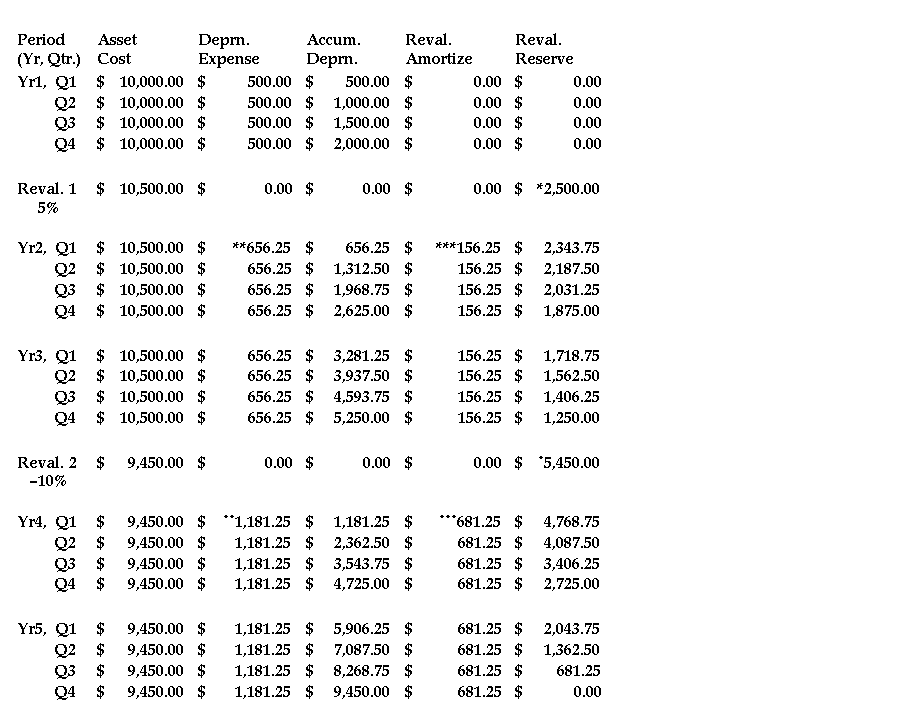#### REVALUATION 1

Year 2, quarter 1, 5% revaluationOracle Assets creates the following journal entries each period to amortize the revaluation reserve: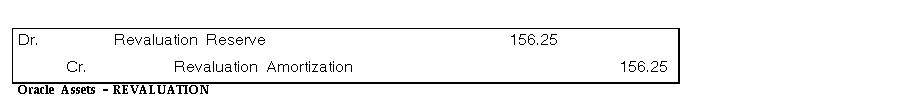#### REVALUATION 2

Year 4, quarter 1, -10% revaluationOracle Assets creates the following journal entries each period to amortize the revaluation reserve: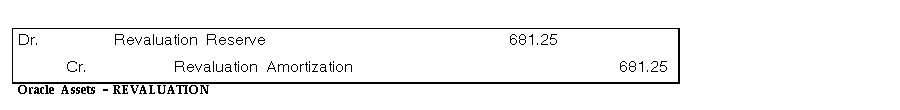### Revaluation of a Fully Reserved Asset

Example 4: You place an asset in service in Year 1, Quarter 1. The asset cost is \$10,000, the life is 5 years, and you are using straight-line depreciation. The asset's life extension factor is 2 and the maximum fully reserved revaluations allowed for this book is 3.

In year 5, quarter 4 the asset is fully reserved. In Year 9, Quarter 1 you want to revalue the asset with a revaluation rate of 5%.

Revaluation Rules:

• Revalue Accumulated Depreciation = Yes
• Amortize Revaluation Reserve = No
First, Oracle Assets checks whether this fully reserved asset has been previously revalued as fully reserved, and that the maximum number of times is not exceeded by this revaluation. Since this asset has not been previously revalued as fully reserved, this revaluation is allowed.

The asset's new revalued cost is \$10,500. The life extension factor for this asset is 2, so the asset's new life is 2 X 5 years = 10 years. Oracle Assets calculates depreciation expense over its new life of 10 years. Oracle Assets calculates the depreciation adjustment of \$2,000 using the new 10 year asset life. It transfers the change in net book value to the revaluation reserve account.

Oracle Assets revalues the accumulated depreciation using the 5% revaluation rate. The change in net book value is transferred to the revaluation reserve account. Since you do not amortize the revaluation reserve, the amount remains in the revaluation reserve account.

The effect of the revaluation is illustrated in the following table: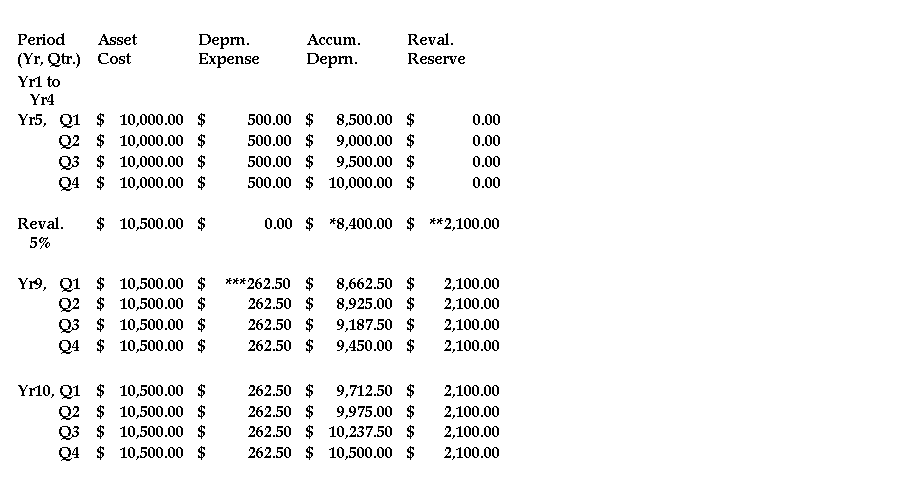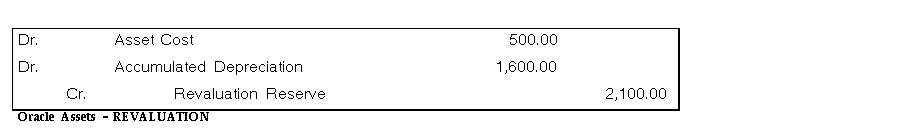### Revaluation with Life Extension Ceiling

Example 5: You place an asset in service in Year 1, Quarter 1. The asset cost is \$10,000, the life is 5 years, and you are using straight-line depreciation. The asset's life extension factor is 3.0 and its life extension ceiling is 2.

In Year 5, Quarter 4 the asset is fully reserved. In year 9, quarter 1 you want to revalue the asset with a revaluation rate of 5%.

Revaluation Rules:

• Revalue Accumulated Depreciation = Yes
• Amortize Revaluation Reserve = No
To determine the depreciation adjustment, Oracle Assets uses the smaller of the life extension factor and the life extension ceiling. Since the life extension ceiling is smaller than the life extension factor, Oracle Assets uses the ceiling to calculate the depreciation adjustment. The new life used to calculate the depreciation adjustment is 2 X 5 years = 10 years, the life extension ceiling of 2 multiplied by the original 5 year life of the asset.

Oracle Assets calculates the asset's depreciation expense under the new life of 10 years up to the revaluation period, and moves the difference between this value and the existing accumulated depreciation from accumulated depreciation to revaluation reserve.

Oracle Assets then determines the new asset cost using the revaluation rate of 5% and revalues the accumulated depreciation with the same rate. Oracle Assets calculates the asset's new life by multiplying the current life by the life extension factor. The asset's new life is 3 X 5 years = 15 years. Oracle Assets bases the new depreciation expense on the revalued net book value and the new 15 year life.

The effect of the revaluation is illustrated in the following table: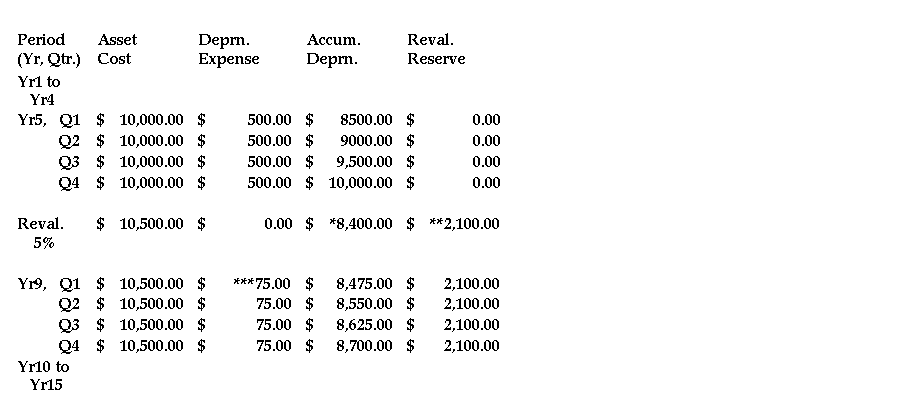Depreciation Adjustment (calculated using life extension ceiling)= 2,000### Revaluation with a Revaluation Ceiling

Example 6: You own an asset which has been damaged during its life. You placed the asset in service in Year 1, quarter 1. The asset cost is \$10,000, the life is 5 years, and you are using straight-line depreciation. You entered a revaluation ceiling of \$10,300 for the asset.

In year 3, quarter 3 you revalue the asset's category with a revaluation rate of 5%.

Revaluation Rules:

• Revalue Accumulated Depreciation = No
• Amortize Revaluation Reserve = Yes
If Oracle Assets applied the new revaluation rate of 5%, the asset's new cost would be higher than the revaluation ceiling for this asset, so instead Oracle Assets uses the ceiling as the new cost. The ceiling creates the same effect as revaluing the asset at a rate of 3%. Oracle Assets bases the asset's new depreciation expense on the revalued asset cost.

The effect of the revaluation is illustrated in the following table: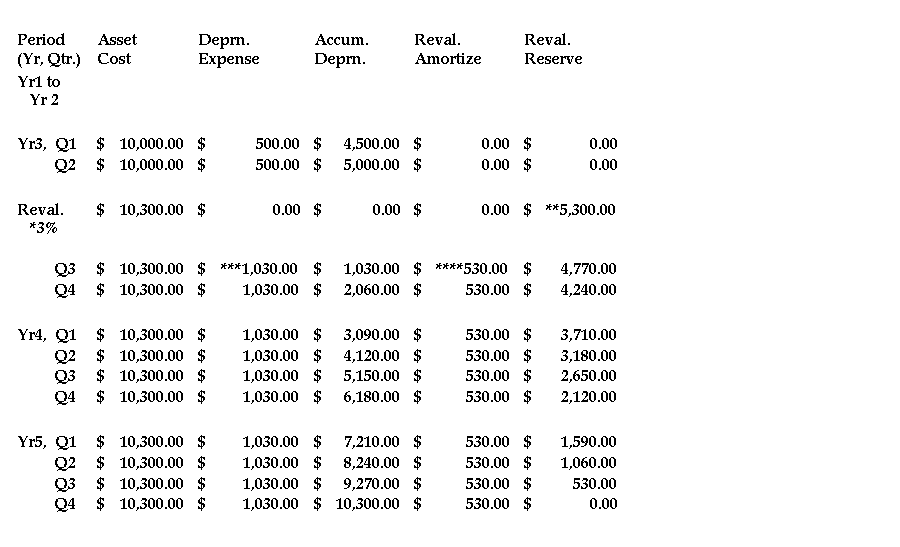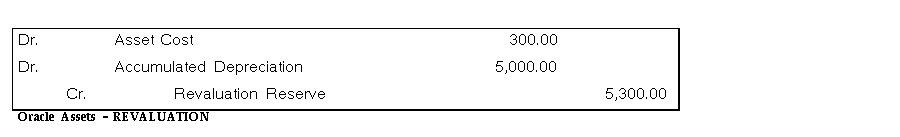Oracle Assets creates the following journal entries each period to amortize the revaluation reserve: